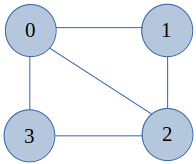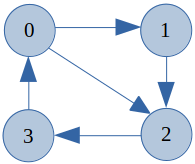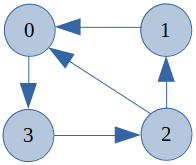×

Search anything:

# Transpose Graph

#### Algorithms Graph Algorithms Data StructuresIn this article, we will explore the idea of Transpose Graph along with the basics of Graph Data Structures. We have presented an algorithm to get the transpose of a graph as well.

## Background on Data Structure

A Data Structure is a process through which a particular data is stored and can be arranged in the disk space of the computer. By doing so, we can easily manipulate the data for future use.
Data Structure are mainly classified into two types,

• Primitive Data Structure
(Example- Int, Char, Float, etc.)
• Abstract Data Structure
(Example- Tree, Stack, Queue, etc.)

Graph is also one of the Data Structure, that falls under type Abstract Data Structure. In this article we'll discuss on Transpose Graph.

## 1. Introduction to Graph

A Graph(`G`) consists of a finite set of Vertices(`V`) and set of Edges(`E`) which connect a pair of nodes. Hence a Graph(`G`) is equivalent to, `G=(V,E)`. Also, Graph is a non-linear Data Structure.##### `Fig a` - Undirected Graph

From the above Graph, the set of Vertices(V) = {0,1,2,3} and set of Edges(E) = {(0,1),(1,2),(2,3),(3,0),(0,2)}.
Graphs are meant to implement the following- `Undirected Graph` and `Directed Graph`.

• In an `Undirected Graph`, The edges(E) are bi-directional or with no direction associated with them. Example- Above diagram(`Fig a`).
• A `Directed Graph` is a set of Vertices connected by Edges and each node having direction associated with it. Below diagram(`Fig b`) represents a Directed Graph,##### `Fig b` - Directed Graph

Let us discuss a bit about `Transpose Graph`. A Transpose Graph is a directed graph in which, It contains the same set of vertices with all of the edges reversed compared to the orientation of the corresponding edges. In other words, consider a Graph(G) which contains Edge(u,v) then Transpose of G will be an Edge(v,u).
Example- For the Graph in `Fig b`, Its Transpose will be as below `Fig c`.##### `Fig c` - Transpose of `Fig b`

As our focus is mainly representation of Transpose Graph, It can be achieved by following two `Graph Representation`,

A two-dimensional matrix `[Row, Column]`, where `Row` represents the source vertices and `Column` represents the destination vertices. And the cost for one edge can be stored between each pair of vertices.
In other words, consider a Directed Graph, `G = (V,E)` and its Transpose will be `G' = (V,E')` is same as Graph(G), instead with arrows reversed.
For Directed Graph, `G != G'`. But for an Undirected graph `G == G'`, reason behind their is no arrow head for edges.

Below is the typical two-dimensional matrix approach to represent the Transpose of Graph,
This is for Graph(G) at `Fig b`

``````    0   1   2   3
----------------
0|  F   T   T   F
1|  F   F   T   F
2|  F   F   F   T
3|  T   F   F   F
``````

This is result of transpose for Graph(G') at `Fig c`

``````    0   1   2   3
----------------
0|  F   F   F   T
1|  T   F   F   F
2|  T   T   F   F
3|  F   F   T   F
``````

From the above example, If their is a Directed edge from vertex u to v then we have marked that respective position as `T`(True) else `F`(False) in the matrix.

• #### Pseudo code

Let us consider a Graph(G) having vertices(V) and edges(E). Here edge's are represented as Row(`i`) and Column(`j`). Following is the pseudo code for the Transpose of Graph(G) where, at the end `G'(i,j)` consists the expected result.

``````for i = 0 to i < V[G]
for j = 0 to j < V[G]
G'(j,i) = G(i,j)
j = j + 1
i = i + 1
``````

A Graph can be represented in List Adjacency in which, Vertices are stored as objects and every vertex stores a list of adjacent vertices.
In this approach it allows the storage of additional data on the vertices. An additional data can be stored if Edges are also stored as objects, in which each vertex stores its incident edges and each edge stores its incident vertices.

• #### Approach

Let consider we have Graph(`G`), which consists of V - Total Vertices to form a Graph(G) and E - Total Edges to form a Graph(G). To find the Transpose of the Graph(`G'`), we need to traverse the adjacency list and as we find a vertex(`v`) in the adjacency list of vertex(`u`) which is the indication that an edge from `u` to `v` in the Graph(G) of Vertices(`V`), we will add an edge from `v` to `u` in its Transpose Graph(`G'`). Hence traversing lists of all Vertices(`V`) from Graph(`G`) we can get the Transpose Graph(`G'`).

• #### Implementation

Consider the below simple implementation of Transpose Graph in Python.

``````# function to add an edge from vertex source(s) to vertex destination(d)

for i in range(v):
print(i, "--> ", end = "")
print()

# function to get Transpose of a graph

# traverse the adjacency list of given graph(G) and for each edge(u, v)
# and add an edge (v, u) in the transpose graph's(G') adjacency list
for i in range(v):

#Program Execution Starts from here

if __name__ == '__main__':

# Vertices of Graph(G)
v = 4
adj = [[] for i in range(v)]

transpose = [[]for i in range(v)]

# displaying adjacency list after transpose
displayGraph(transpose, v)
``````

## Output

``````# 0 --> 3
# 1 --> 0
# 2 --> 0 1
# 3 --> 2
``````

## 4. Conclusion

As we have discussed about the basics of the following-
Data Structure, Graph (Directed and Undirected), Representing a Graph in List and Matrix Adjacency. Most of the times, by common practice in industry they make use of List Adjacency to solve real time problem.
Due to the reason that list representation also stores the address along with the data of the particular instance of edge during traversal.#### Shiva Basava P

Shiva is a Senior Analyst/ Software Engineer at Capgemini Technology Services India Limited and has been a Software Developer, Intern at OPENGENUS